### IMO Shortlist 2005 problem G2

Kvaliteta:
Avg: 3.0
Težina:
Avg: 6.0
Six points are chosen on the sides of an equilateral triangle$ABC$: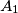$A_1$,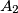$A_2$ on$BC$,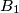$B_1$,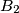$B_2$ on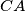$CA$ and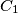$C_1$,$C_2$ on$AB$, such that they are the vertices of a convex hexagon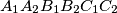$A_1A_2B_1B_2C_1C_2$ with equal side lengths.

Prove that the lines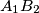$A_1B_2$,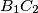$B_1C_2$ and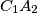$C_1A_2$ are concurrent.

Bogdan Enescu, Romania
Source: Međunarodna matematička olimpijada, shortlist 2005